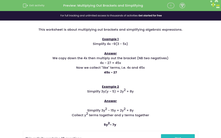# Expand Single Brackets and Simplify

In this worksheet, students will multiply out brackets and simplify algebraic expressions.Key stage:  KS 3

Curriculum topic:   Algebra

Curriculum subtopic:   Simplify Algebraic Expressions to Maintain Equivalence

Popular topics:   Algebra worksheets

Difficulty level:#### Worksheet Overview

This activity is about expanding (multiplying out brackets) and simplifying algebraic expressions.

Example 1

Simplify 4x - 9(3 - 5x)

We copy down the 4x then multiply out the bracket:

4x - 27 + 45x (Did you spot that -9 x 3 = a negative 27, but -9 x -5x = a positive 45x?)

Now we collect like terms, i.e. 4x + 45x

49x - 27

Example 2

Simplify 3y(y - 5) + 2y2 + 8y

First, start by expanding the bracket 3y(y - 5) to get 3y2 - 15y

Simplify 3y2 - 15y + 2y2 + 8y

Collect y2 terms together and y terms together

5y- 7y

Right, let's have a go at some questions now.

### What is EdPlace?

We're your National Curriculum aligned online education content provider helping each child succeed in English, maths and science from year 1 to GCSE. With an EdPlace account you’ll be able to track and measure progress, helping each child achieve their best. We build confidence and attainment by personalising each child’s learning at a level that suits them.

Get started